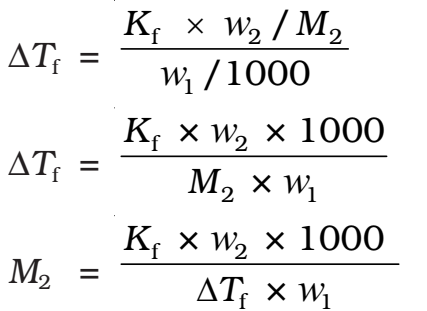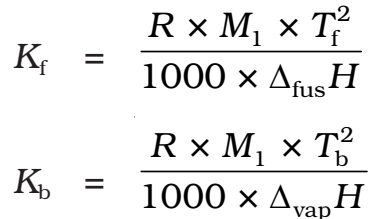# Colligative Properties

Colligative: from Latin. = Co mean ‘together’; ligare means ‘to bind’.

Colligative properties are those properties which depend only upon the number of solute particles in a solution irrespective of their nature.

## Relative Lowering of Vapour Pressure:

It is the ratio of lowering in the vapour to vapour pressure of the pure solvent. The relative lowering of the vapour pressure of the solution containing a nonvolatile solute is equal to the mole fraction of solute in the solution.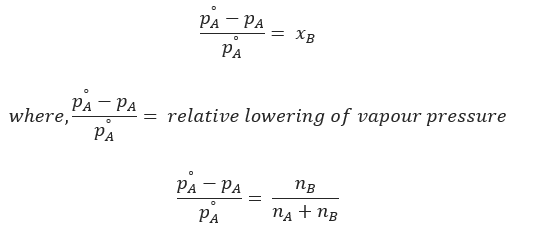For dilute solution, nB << nA . Hence,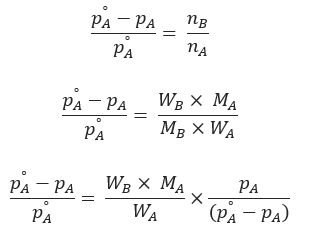Above expression is used to find the molecular weight of an unknown solute dissolved in a given solvent. Where, WB and WA = mass of solute and solvent respectively. MB and MA = molecular weight of solute and solvent respectively.

Ostwald and walker method is used to determine the relative lowering of vapour pressure.

## Elevation in Boiling Point (∆Tb)

Boiling point of a liquid is the temperature at which its vapour pressure is equal to the atmospheric pressure. As the vapour pressure of a solution containing a nonvolatile solute is lower than that of the pure solvent, it boiling point will be higher than that of the pure solvent as shown in figure. The increase in boiling point is known as elevation in boiling point, ∆Tb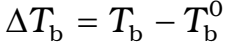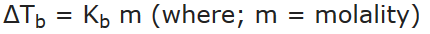Kb is molal elevation constant or ebullioscopic constant. Molecular mass of solute can be calculated as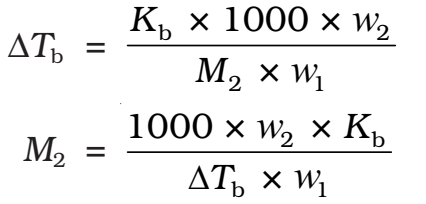Thus, in order to determine M2, molar mass of the solute, known mass of solute in a known mass of the solvent is taken and ΔTb is determined experimentally for a known solvent whose Kb value is known.

∆Tb = Kb × m = 0.52 K kg mol-1 × 0.1 mol kg-1 = 0.052 K

Since water boils at 373.15 K at 1.013 bar pressure, therefore, the boiling point of solution will be 373.15 + 0.052 = 373.202 K.

The boiling point elevation of a solution is determined by

1. Landsberger’s method
2. Cottrell’s method

## Depression in Freezing Point (ΔTf)

The freezing point of a liquid is the temperature at which its vapour pressure of the solvent in its liquid and solid phase become equal. As we know that vapour pressure of a solution containing nonvolatile solute is lower than that of the pure solvent, the solid form gets separated out at a lower temperature as shown in the figure.